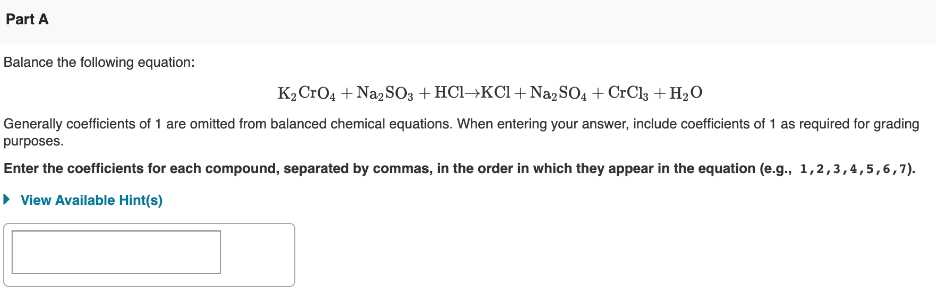# Balance the following equation: K2CrO4 + Na2SO3 + HCl → KCl + Na2SO4 + CrCl3 + H2O Generally coefficients of 1 are omitted from balanced chemical equations. When entering your answer, include coefficients of 1 as required for grading purposes. Enter the coefficients for each compound, separated by commas, in the order in which they appear in the equation (e.g., 1, 2, 3, 4, 5, 6, 7).